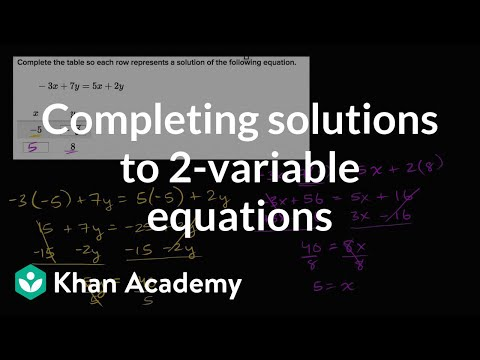Video

# Systems of equations with elimination: x + 2y = 6 and 4x - 2y = 14 (Full video)

Description: Sal demonstrates how to solve the system of equations x + 2y = 6 and 4x - 2y = 14 using the elimination method. And they gave us two equations here: x plus 2y is equal to 6 and 4x minus 2y is equal to 14. So if I have x plus 2y is equal to 6, you learned early on in algebra that you can manipulate this equation in any way as long as whatever you do to the left-hand side of the equation, you do the right-hand side. If this is equal to that, the only way that the equality will still hold is whatever you do to this you also do to the right-hand side.

### Other videos you might be interested in### Solving Systems of Equations by Substitution (Full video)

#### Student Achievement Partners